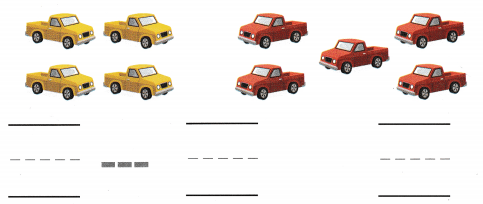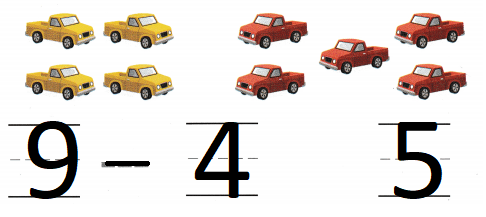# Texas Go Math Kindergarten Module 10 Assessment Answer Key

Refer to our Texas Go Math Kindergarten Answer Key Pdf to score good marks in the exams. Test yourself by practicing the problems from Texas Go Math Kindergarten Module 10 Assessment Answer Key.

## Texas Go Math Kindergarten Module 10 Assessment Answer Key

Concepts and Skills

Question 1.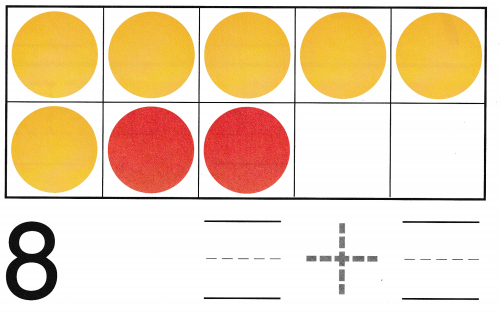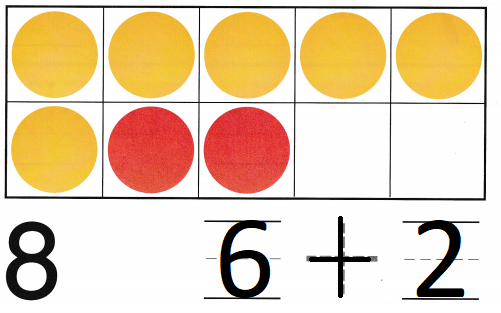Explanation:
Number of yellow counters are 6
Number of red counters are 2
total number of counters are 8
6 + 2 = 8

Question 2.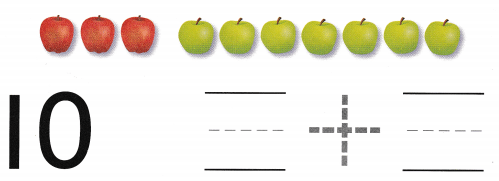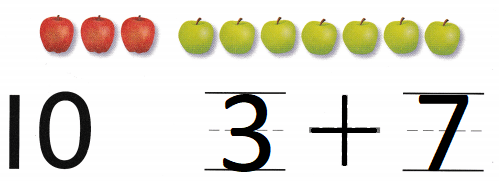Explanation:
There are 3 red apples
and there are 7 green apples
3 + 7 = 10
so, 10 apples in all.

Directions
1. Count the counters in the ten frame. Write the number pair that makes 8. 2. Look at the picture. Write the number pair that makes 10. TEKS K.2.I

Question 3.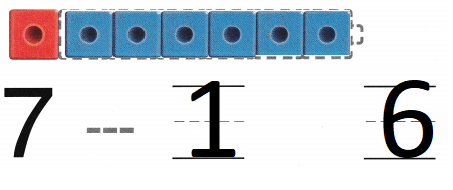Explanation:
Number of red cubes are 1
total number of cubes are 7
7 – 1 = 6
number of blue cubes are 6

Question 4.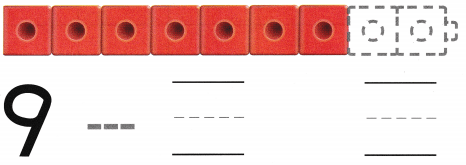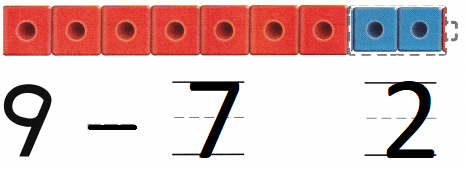Explanation:
Number of red cubes are 7
total number of cubes are 9
9 – 7 = 2
number of blue cubes are 2

Question 5.
Texas Test Prep